null Adversarial Auto-Encoders - K码农

• Distribution of hidden code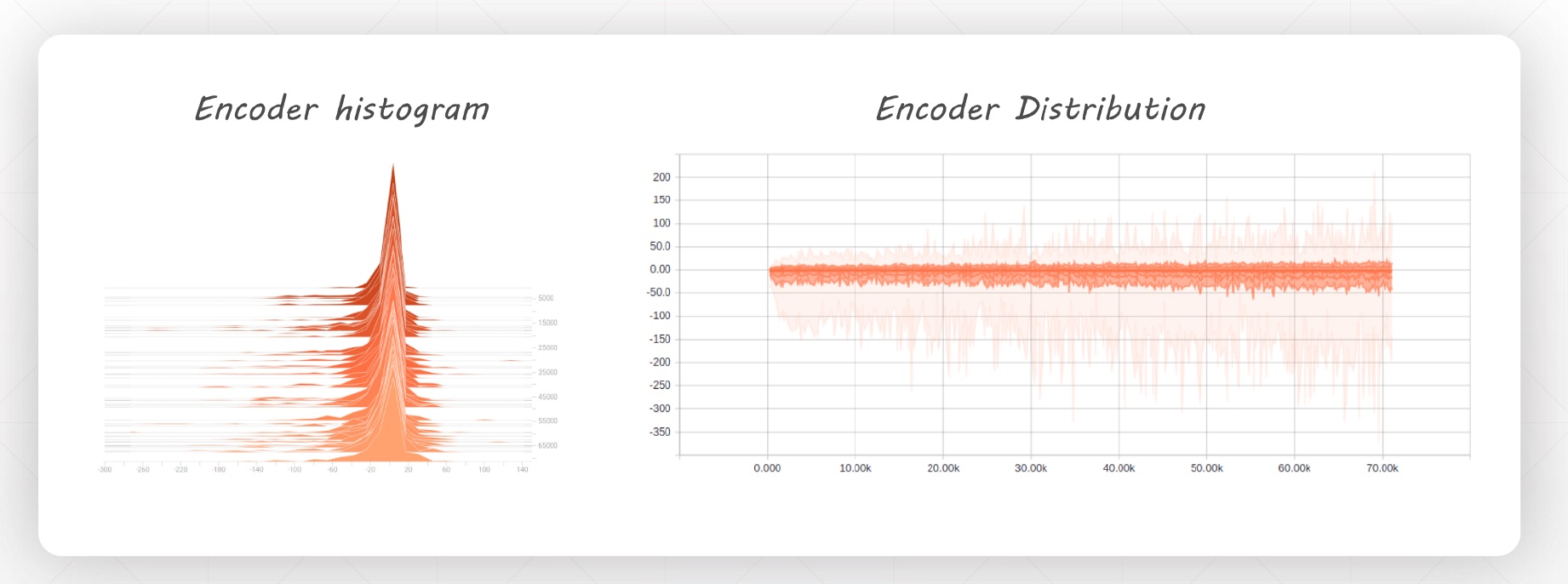• Give more details after GAN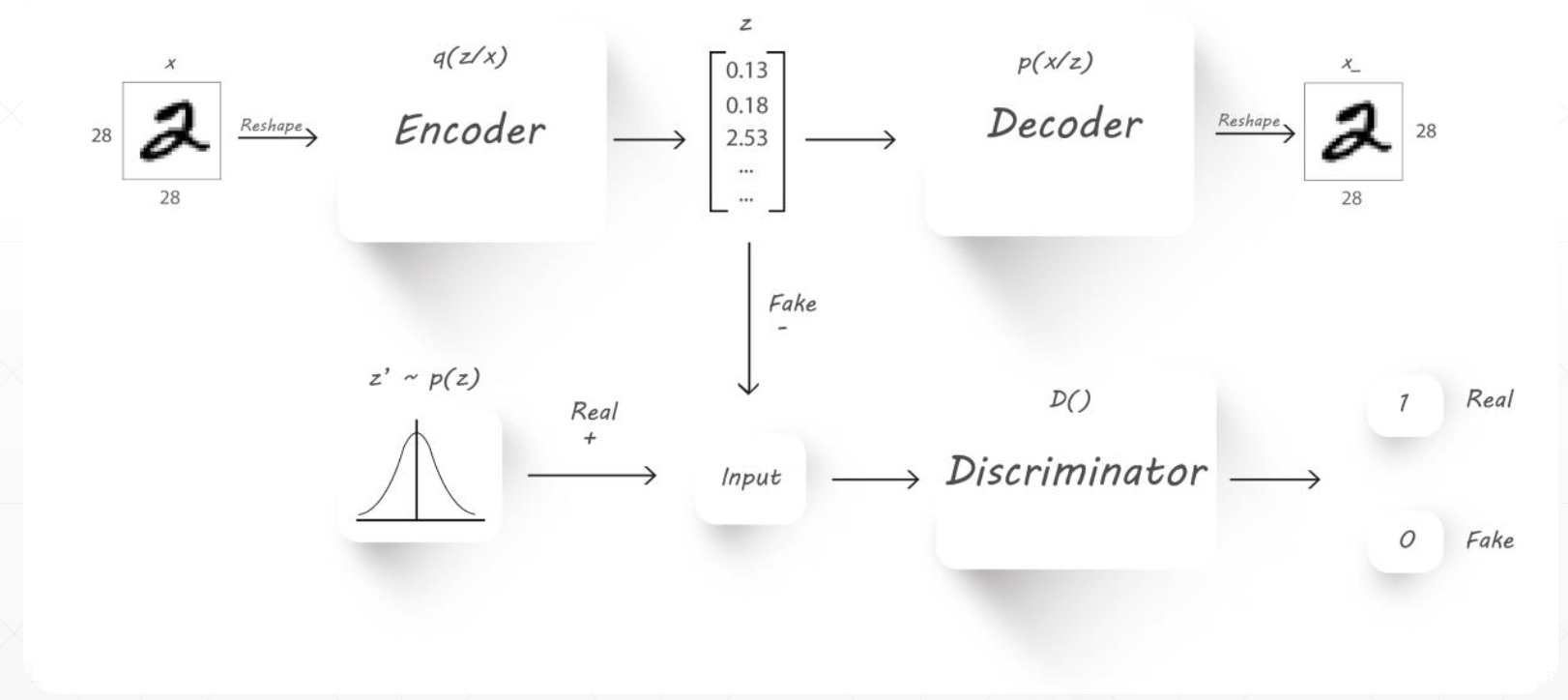# Another Approach: q(z)->p(z)

• Explicitly enforce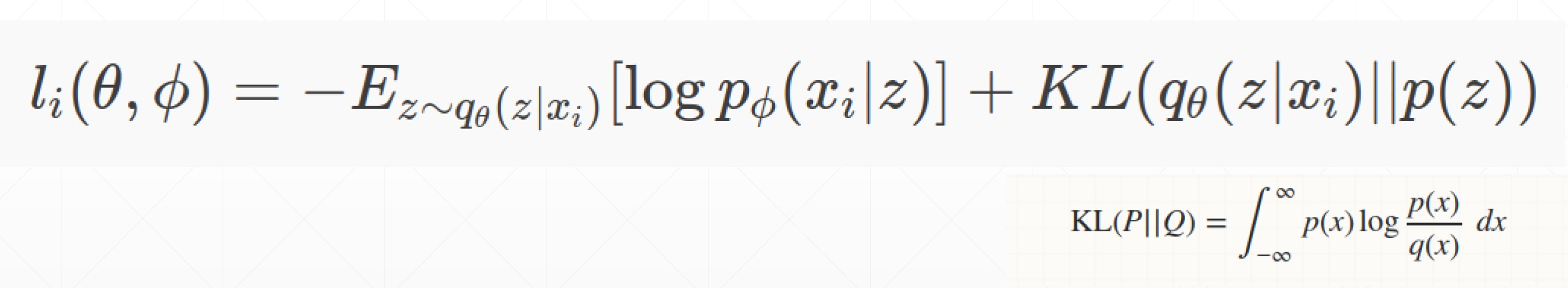## Intuitively comprehend KL(p|q)

$KL\in \left[0,+\mathrm{\infty }\right]$$KL \in{[0,+\infty]}$

• KL = 0 --> $p\approx q$$p\approx{q}$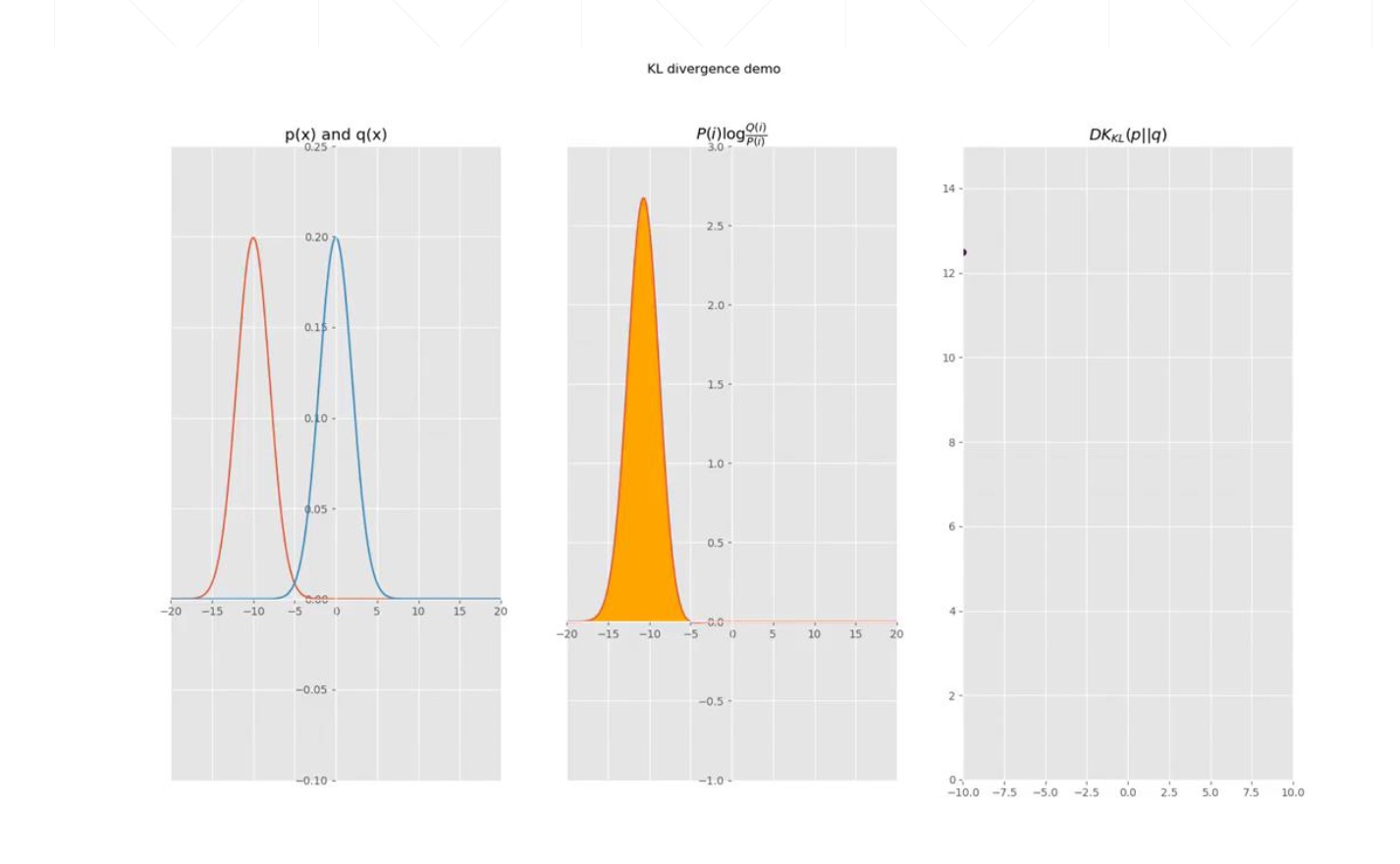# Minimize KL Divergence

• Evidence Lower BOund

$KL\left({q}_{\theta }\left(z|{x}_{i}\right)||p\left(z\right)\right)$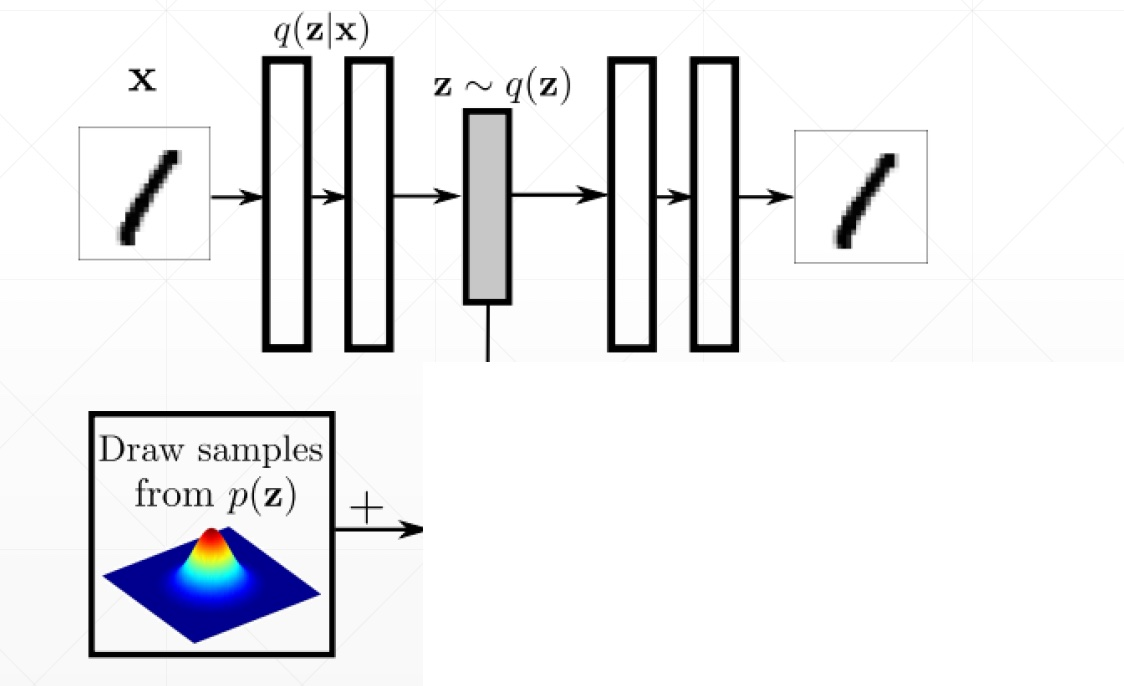## How to compute KL between q(z) and p(z)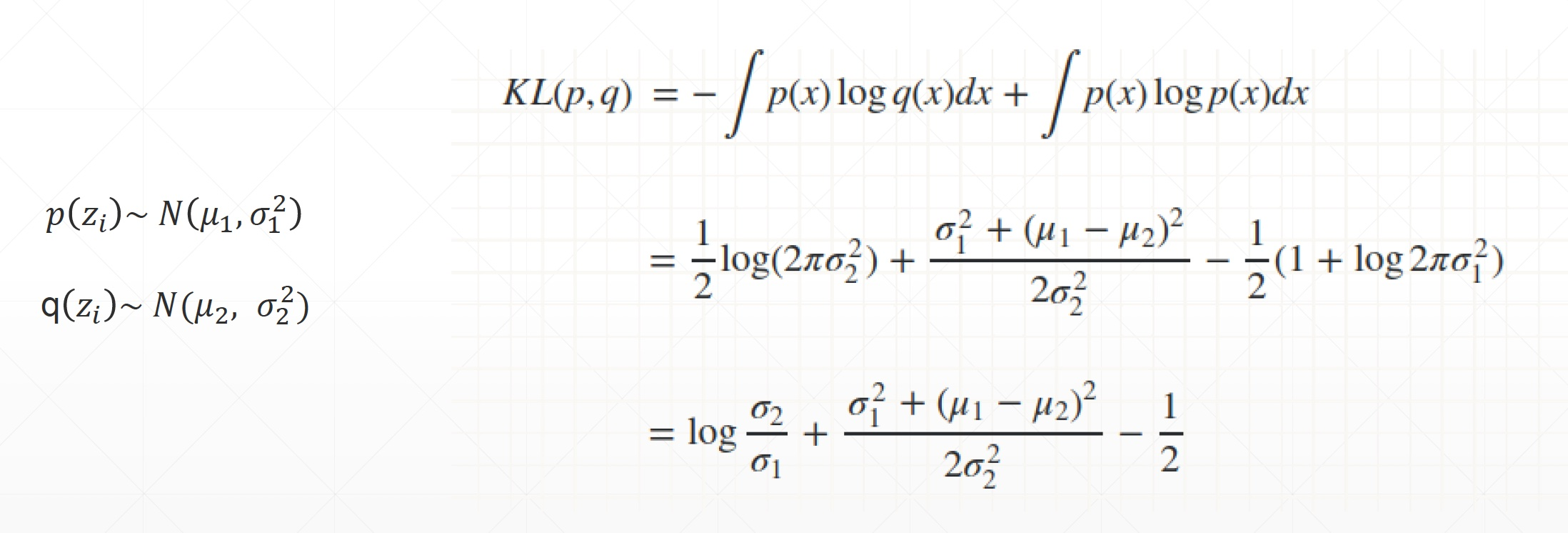• 重建变量
• 隐藏变量逼近p的分布